Question

# Exercise 1 Consider the two matrices o 3 1 0 ) Say whether the following matrix...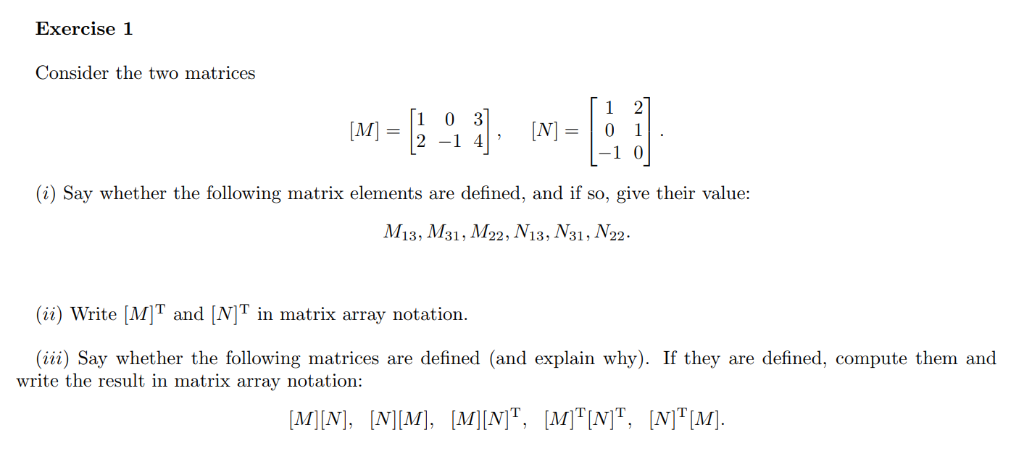Exercise 1 Consider the two matrices o 3 1 0 ) Say whether the following matrix elements are defined, and if so, give their value: 13, 1M31,M22, V13, V31, /V22 (i) Write MIT and [NI in matrix array notation. (iii) Say whether the following matrices are defined (and explain why). If they are defined, compute them and write the result in matrix array notation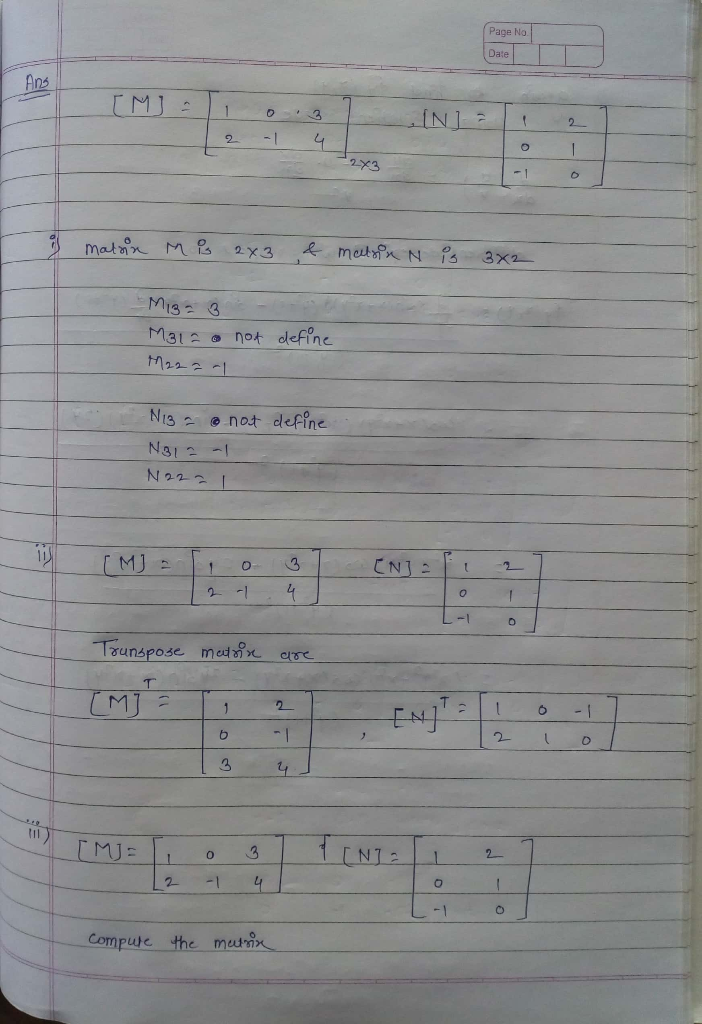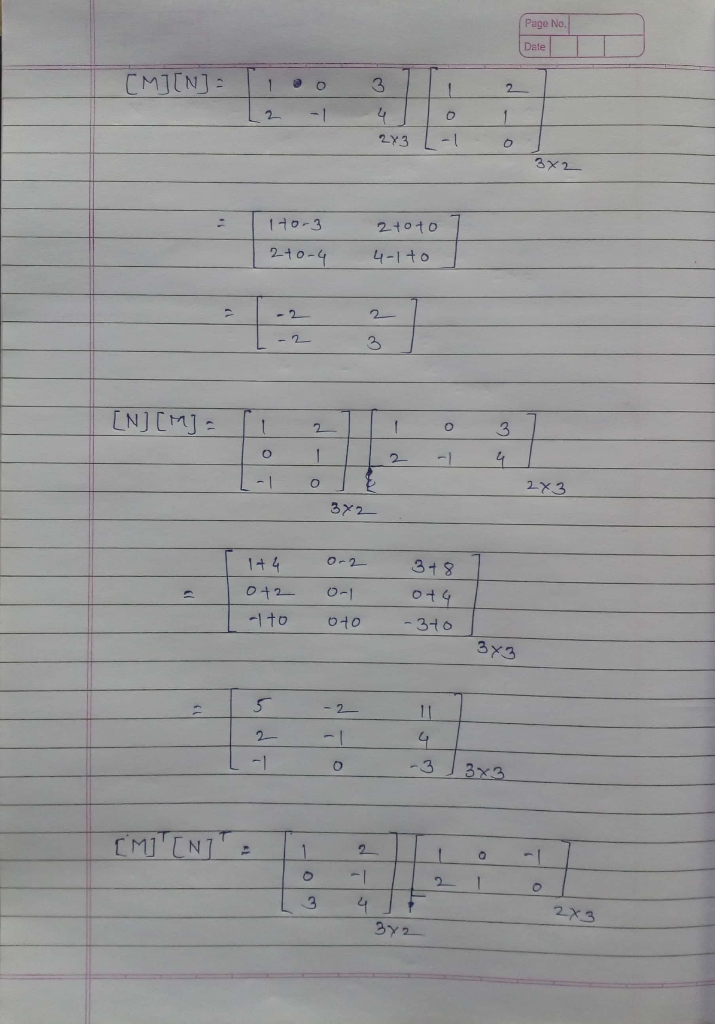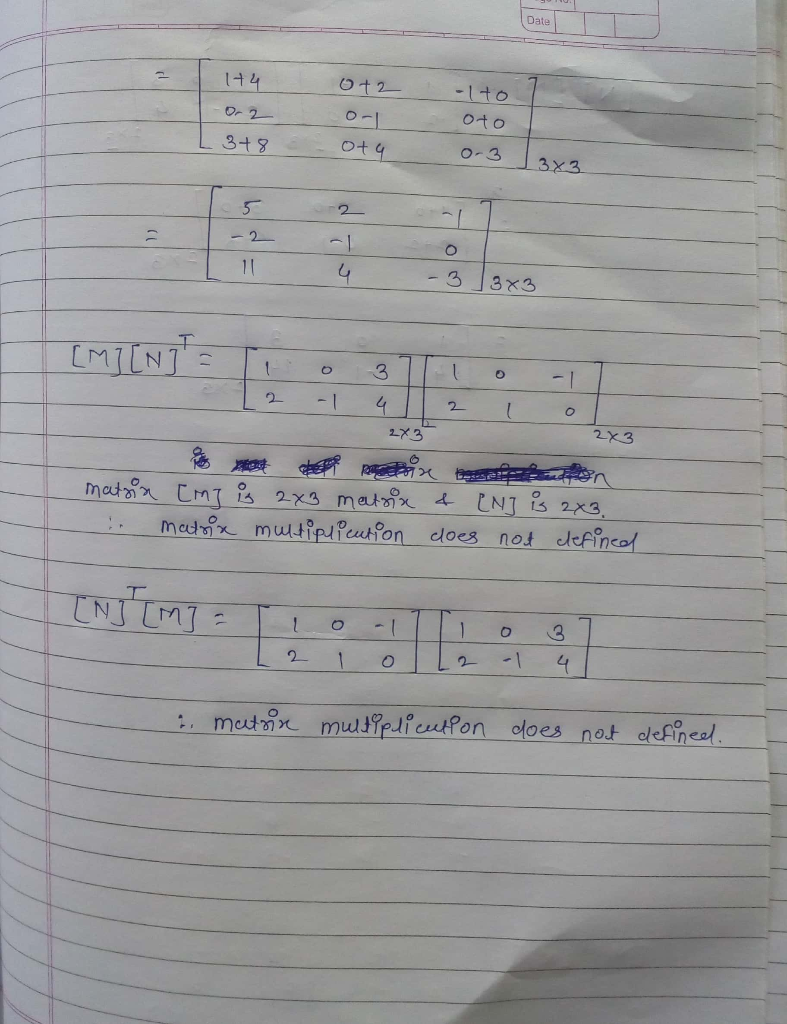#### Earn Coins

Coins can be redeemed for fabulous gifts.

Similar Homework Help Questions
• ### Algebra of matrices. 3. (a) If A is a square matrix, what does it mean to say that B is an inverse of A (b) Define AT....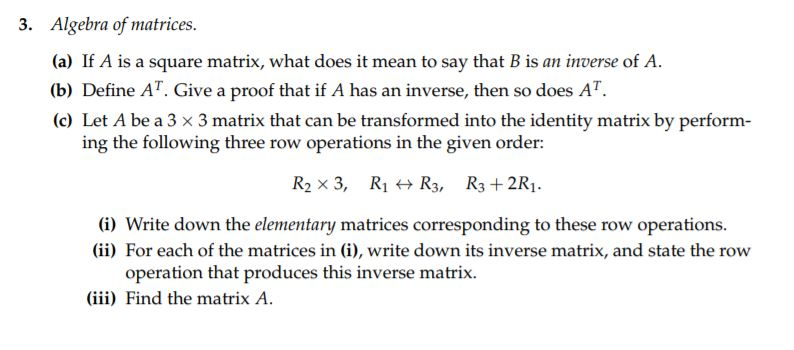Algebra of matrices. 3. (a) If A is a square matrix, what does it mean to say that B is an inverse of A (b) Define AT. Give a proof that if A has an inverse, then so does AT. (c) Let A be a 3 x 3 matrix that can be transformed into the identity matrix by perform ing the following three row operations in the given order: R2 x 3, Ri R3, R3+2R1 (i) Write down the elementary...

• ### Compute each of the following matrix multiplications or say why it is impossible. 1 2[2 3 1-3 4 0 4 1 3 -1 6 (iii) [0-3...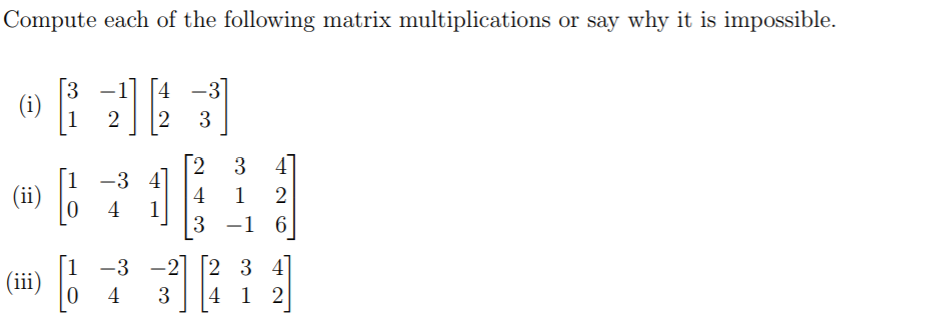Compute each of the following matrix multiplications or say why it is impossible. 1 2[2 3 1-3 4 0 4 1 3 -1 6 (iii) [0-33][2 33] 0 4 34 1 2 Compute each of the following matrix multiplications or say why it is impossible. 1 2[2 3 1-3 4 0 4 1 3 -1 6 (iii) [0-33][2 33] 0 4 34 1 2

• ### 2. Partitioned matrices A matrix A is a (2 x 2) block matrix if it is...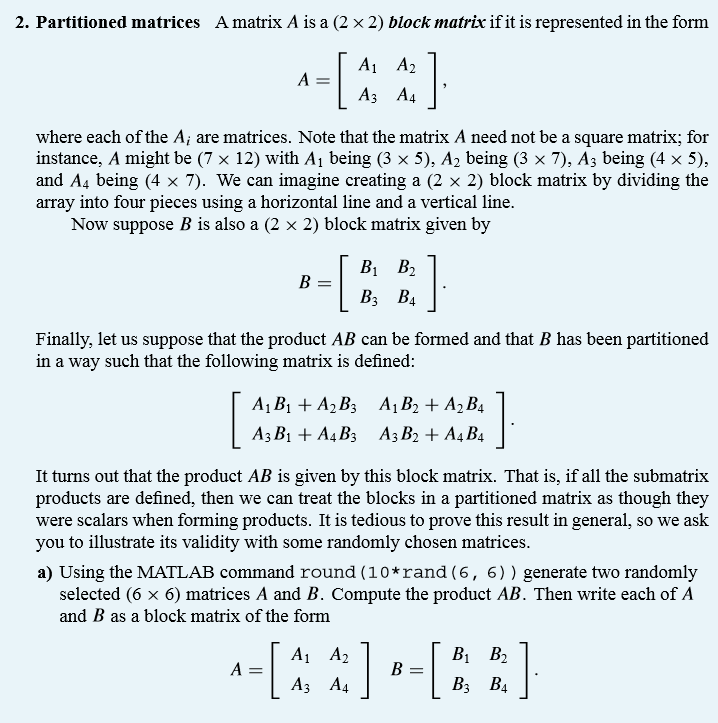2. Partitioned matrices A matrix A is a (2 x 2) block matrix if it is represented in the form [ A 1 A2 1 A = | A3 A4 where each of the A; are matrices. Note that the matrix A need not be a square matrix; for instance, A might be (7 x 12) with Aj being (3 x 5), A2 being (3 x 7), A3 being (4 x 5), and A4 being (4 x 7). We can...

• ### Matrix Methods/Linear Algebra: Please show all work and justify the answer! 1. Consider the following matrices....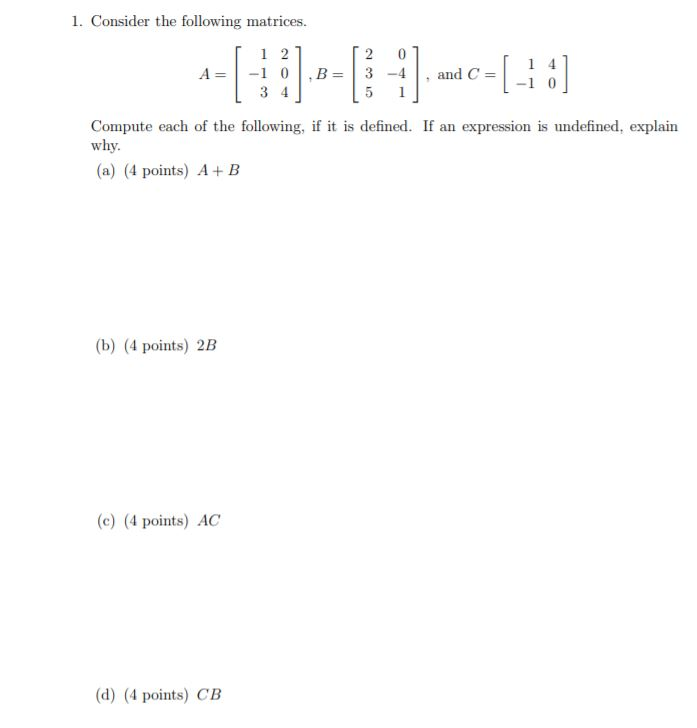Matrix Methods/Linear Algebra: Please show all work and justify the answer! 1. Consider the following matrices. [-1:] 1 2 2 0 A= -10.B=3-4 and C= 3 4 5 Compute each of the following, if it is defined. If an expression is undefined, explain why. (a) (4 points) A+B (b) (4 points) 2B (e) (4 points) AC (d) (4 points) CB

• ### 1. Consider the following matrices. A= 1 2 -1 0 3 4 B 2 3-4 5...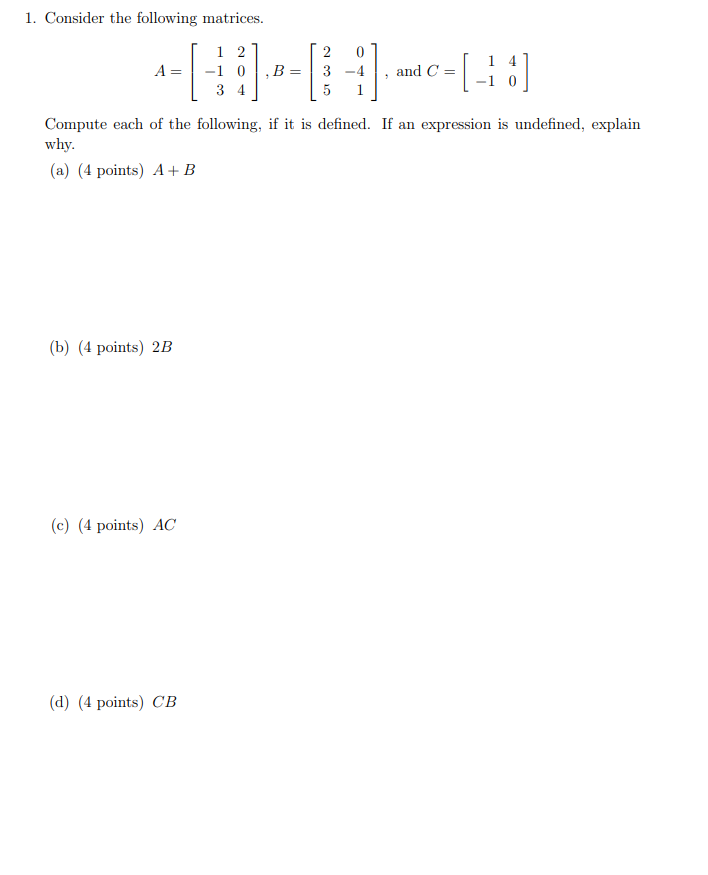1. Consider the following matrices. A= 1 2 -1 0 3 4 B 2 3-4 5 1 and C= -[-1:] Compute each of the following, if it is defined. If an expression is undefined, explain why. (a) (4 points) A+B (b) (4 points) 2B (c) (4 points) AC (d) (4 points) CB

• ### 1 Compute and completely factor the characteristic polynomial of the following matrix: 0 A= -4 5...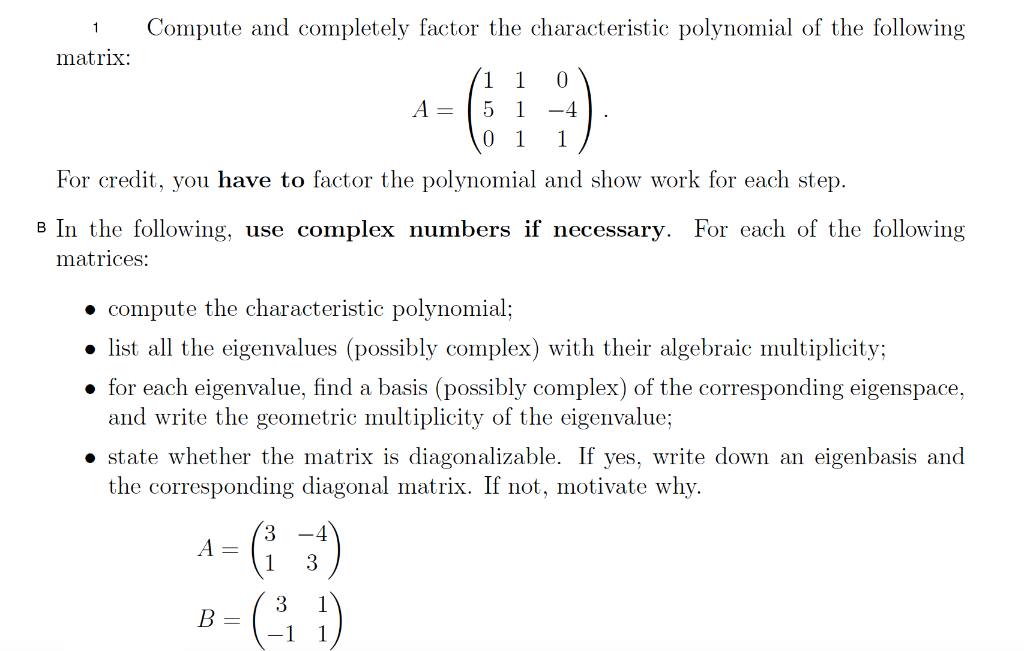1 Compute and completely factor the characteristic polynomial of the following matrix: 0 A= -4 5 0 1 1 For credit, you have to factor the polynomial and show work for each step. B In the following, use complex numbers if necessary. For each of the following matrices: • compute the characteristic polynomial; • list all the eigenvalues (possibly complex) with their algebraic multiplicity; • for each eigenvalue, find a basis (possibly complex) of the corresponding eigenspace, and write the...

• ### 5. Consider the matrix A-1-6-7-3 Hint: The characteristic polynomial of A is p(λ ) =-(-2)0+ 1)2....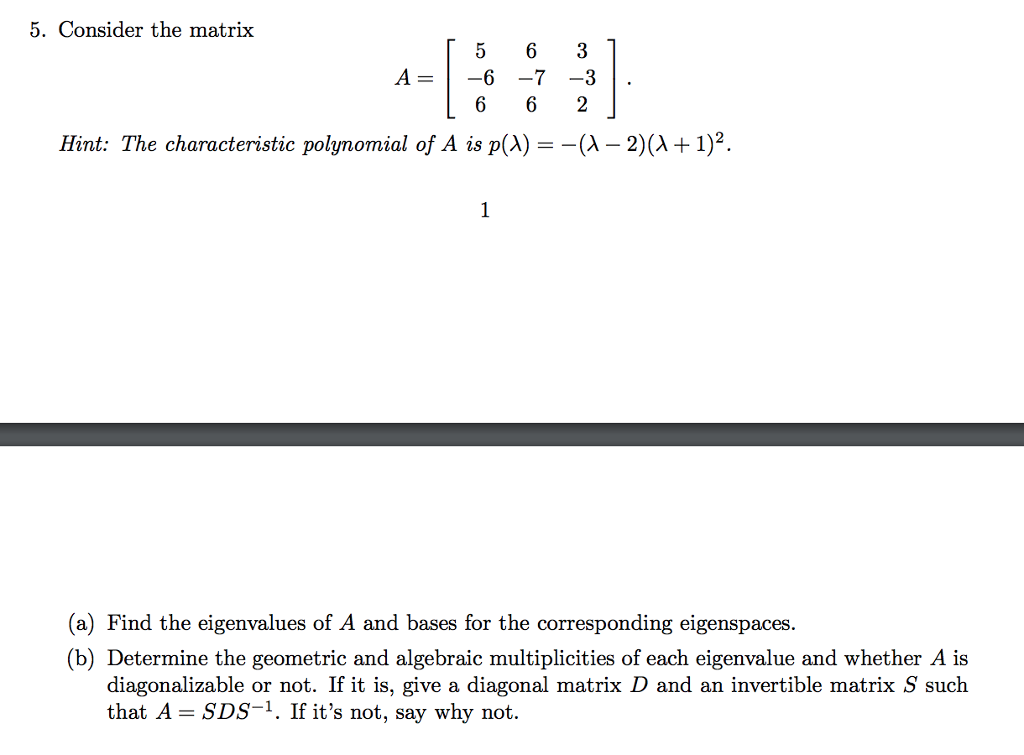5. Consider the matrix A-1-6-7-3 Hint: The characteristic polynomial of A is p(λ ) =-(-2)0+ 1)2. (a) Find the eigenvalues of A and bases for the corresponding eigenspaces. (b) Determine the geometric and algebraic multiplicities of each eigenvalue and whether A is diagonalizable or not. If it is, give a diagonal matrix D and an invertible matrix S such that A-SDS-1. If it's not, say why not.

• ### solve and please write explanation as well. 2. Consider the following matrices: [1 2 1-1 3...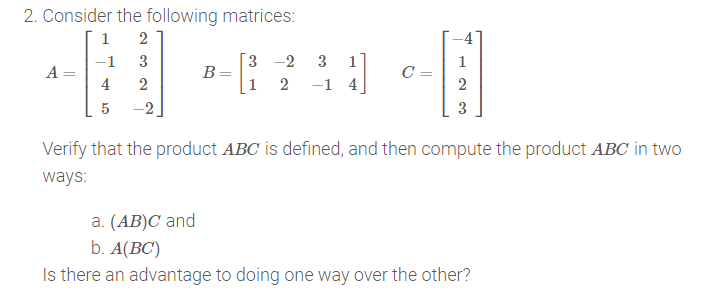solve and please write explanation as well. 2. Consider the following matrices: [1 2 1-1 3 (3 -2 A= 4 2 (1 2 [ 5 -2 3 -1 4] CON Verify that the product ABC is defined, and then compute the product ABC in two ways: a. (AB)C and b. A(BC) Is there an advantage to doing one way over the other?

• ### Matlab answer only Form 1 6) [10 pts] Consider the following two matrices B=[S A 3...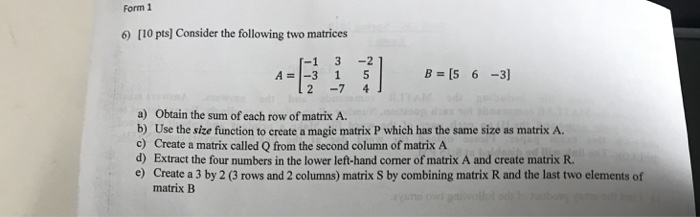Matlab answer only Form 1 6) [10 pts] Consider the following two matrices B=[S A 3 1 5 -7 4 6-3] a) Obtain the sum of each row of matrix A. b) Use the size function to create a magic matrix P which has the same size as matrix A. c) Create a matrix called Q from the second column of matrix A d) Extract the four numbers in the lower left-hand corner of matrix A and create matrix R....

• ### Write a c++ program: Many mathematical problems require the addition, subtraction, and multiplication of two matrices. Write an ADT Matrix. You may use the following class definition: const int MAX_RO...

Write a c++ program: Many mathematical problems require the addition, subtraction, and multiplication of two matrices. Write an ADT Matrix. You may use the following class definition: const int MAX_ROWS = 10; const int MAX_COLS = 10; class MatrixType { public: MatrixType(); void MakeEmpty(); void SetSize(int rowsSize, int colSize); void StoreItem(int item, int row, int col); void Add(MatrixType otherOperand, MatrixType& result); void Sub(MatrixType otherOperand, MatrixType& result); void Mult(MatrixType otherOperand, MatrixType& result); void Print(ofstream& outfile); bool AddSubCompatible(MatrixType otherOperand); bool MultCompatible(MatrixType otherOperand);...